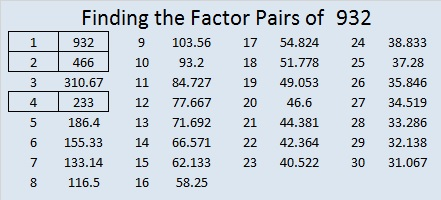# 932 and Level 1

These sixteen clues are all you need to solve this puzzle. First, figure out where the factors from 1-10 go in the first column and the top row so that clues in the puzzle and the factors will be like a multiplication table. After you write in all the factors, you can decide if you’d like to fill in the rest of the table. It’s not difficult.Print the puzzles or type the solution on this excel file: 10-factors-932-941

Now here’s a few facts about the number 932:

26² + 16² = 932
That means 420² + 832² = 932²

Why? Because 420-832-932 can be calculated from 26² – 16², 2(26)(16), 26² + 16²

• 932 is a composite number.
• Prime factorization: 932 = 2 × 2 × 233, which can be written 932 = 2² × 233
• The exponents in the prime factorization are 2 and 1. Adding one to each and multiplying we get (2 + 1)(1 + 1) = 3 × 2  = 6. Therefore 932 has exactly 6 factors.
• Factors of 932: 1, 2, 4, 233, 466, 932
• Factor pairs: 932 = 1 × 932, 2 × 466, or 4 × 233
• Taking the factor pair with the largest square number factor, we get √932 = (√4)(√233) = 2√233 ≈ 30.528675This site uses Akismet to reduce spam. Learn how your comment data is processed.